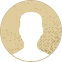Top Special Offer! Check discount

Get 13% off your first order - useTopStart13discount code now!

# Linear regression

186 views 2 pages ~ 371 words
Get a Custom Essay Writer Just For You!

Experts in this subject field are ready to write an original essay following your instructions to the dot!

The effect of GPA on Post-MBA wages will be examined in the research hypothesis. The following are the null and alternative hypotheses:

H0: B1 = 0\sH1: B1 ≠ 0

Post-MBA wages will be the dependent variable, and GPA will be the independent variable. In other words, the research topic looks into how obtaining a GPA affects post-MBA wages.

The outcomes of regression

When salaries (dependent variables) were regressed against GPA (independent variable) using IBM SPSS, the following results were obtained.

Summary of the Model

R R Square Adjusted R Square Std. Estimate Change Statistics Error

Change R Square Change F Change df1 df2 Sig. F Change

1

.048a

.002

-.009

1.260

.002

.205

1

87

.652

a. Predictors: (Constant), GPA

ANOVAa

Model

Sum of Squares

df

Mean Square

F

Sig.

1

Regression

.326

1

.326

.205

.652b

Residual

138.146

87

1.588

Total

138.472

88

a. Dependent Variable: Salaries

b. Predictors: (Constant), GPA

Coefficientsa

Model

Unstandardized Coefficients

Standardized Coefficients

t

Sig.

95.0% Confidence Interval for B

B

Std. Error

Beta

Lower Bound

Upper Bound

1

(Constant)

1.750

.933

1.875

.064

-.105

3.605

GPA

.118

.260

.048

.453

.652

-.399

.634

a. Dependent Variable: Salaries

Interpreting and Reporting the Output of Regression Analysis

Model Summary

The model summary table has the “R” value, which measures the quality of prediction of the dependent variable (Salaries). The value of R is 0.048 from the model summary table. The R-squared is the coefficient of determination, in other words, the proportion of variance in the dependent variable. In our case, it is 0.002, signifying that 0.2% of the variability of the dependent variable salaries is explained by the independent variable. The important value is the adjusted R-squared which -0.009.

Statistical significance

The ANOVA table shows F-ratio which test if the regression model is a good fir of the data. The F (1, 87) value is 0.205 but the p-value is 0.652. since the p-value is greater than 0.05, the regression model is not a good fir of the data.

Estimated model coefficients

We use the Coefficients table can be used to form a general equation of the relationship between Salaries and GPA. The equation is

Salaries = 1.72 + 0.118*(GPA)

Using the above analysis, we can conclude that we have rejected the null hypothesis (H0: B1 = 0) since our constant B1 = 0.118.

NB: I had to combine the SR50000999 – SR25000029999 into one variable which I have named it SR[salaries]. However, I realized that the new variable SR was exact to the variable “MonthlybasesalariesbeforetheMBA”

April 06, 2023
Category:
Subcategory:
Subject area:
Number of pages

2

Number of words

371

52Rate:

4.8

Expertise Model
Verified writer

Cruz021 has helped me with my personal statement essay. I had some ideas that I wrote down for my assigned writer. I received an excellent two-page paper that had my vision followed the best way possible.

Hire Writer

This sample could have been used by your fellow student... Get your own unique essay on any topic and submit it by the deadline.

Eliminate the stress of Research and Writing!

Hire one of our experts to create a completely original paper even in 3 hours!